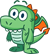# Function datasets

Function datasets are created as an output of Create Regression Model. A function dataset contains the equation and statistics of a regression model.

## Use a function dataset

Function datasets are used as the input regression model for Predict Variable. You can open Predict Variable by dragging a function dataset to a map card.

A point chart showing the coefficients and confidence intervals for the intercept and each explanatory variable can be created by expanding a function dataset in the data pane and clicking View confidence intervals.

##### Tip:Drag a function dataset onto the point chart created from a different regression model to compare the confidence intervals for the explanatory variables between models.

## Statistics

Function datasets store the equation and statistics from a regression model. Statistics can be viewed by expanding the function dataset in the data pane or by opening the data table.

The following statistics are available in the data pane:

StatisticDescription

Regression equation

The regression equation is in the following format:

``y=b0+b1x1+b2x2+...+bnxn``

where y is the dependent variable, bn represents the calculated parameters, and xn represents the explanatory variables.

R2

The R2 value, also known as the coefficient of determination, is a number between 0 and 1 that measures how well the line of best fit models the data points, with values closer to 1 indicating more accurate models.

Adjusted R2 is also a measure between 0 and 1, but it accounts for more additional predictors that may cause a better fit in a model based on chance alone. Therefore, it is best to use the Adjusted R2 value when the model has a large number of predictors, or when comparing models with different numbers of predictors.

Durbin-Watson

The Durbin-Watson test measures autocorrelation in residuals from a regression analysis on a scale of 0 to 4. On this scale, 0 to 2 is positive autocorrelation, 2 is no autocorrelation, and 2 to 4 is negative autocorrelation. It is best to have low autocorrelation in a regression model, meaning Durbin-Watson test values closest to 2 are more favorable.

##### Note:

The Durbin-Watson test calculation is dependent on the order of your data. It is important that your data be ordered sequentially, especially if the data is related to time. If your data is not ordered properly, then the value of the Durbin-Watson test may not be accurate.

Residual standard error

The residual standard error measures the accuracy with which the regression model can predict values with new data. Smaller values indicate a more accurate model. The value of the residual degrees of freedom is also given with the residual standard error.

F statistic

The F statistic is used to determine the predictive capability of your regression model by determining if the coefficients are significantly different from 0. The F statistic is given as a value greater than or equal to 0 and includes two values for degrees of freedom, the first being the degrees of freedom for explanatory variables, and the second being the degrees of freedom for the residuals.

p-value

The p-value for the F statistic is a test of global significance for your regression model. A p-value is given as a value between 0.0 and 1.0. Values between 0 and 0.05 indicate that your global model is statistically significant.

The following statistics are available in the data table:

StatisticDescription

Variable

The intercept and the names of the explanatory variables.

Coefficient

The b-values for the regression equation, which correspond to the y-intercept and the slope for each explanatory variable.

Standard error

The standard error measures the variation in each of the predictors used in the model. Smaller values indicate more accurate predictors.

t-value

The t-value is used to determine the predictive capability of each regression coefficient by determining if the coefficients are significantly different from 0.

p-value

The p-value is related to the t-value and tests local significance for the coefficients in your regression model. A p-value is given as a value between 0.0 and 1.0. Values between 0.0 and 0.05 indicate that the coefficient is statistically significant.

Confidence interval

Confidence intervals give the upper and lower limits within which you can have a certain degree of certainty that the coefficient falls within the range. For example, if the lower 95 percent confidence interval is 10 and the upper 95 percent confidence interval is 15, you can have 95 percent confidence that the true value of the coefficient is between 10 and 15.

The following confidence intervals are given in the data table:

• Lower 90 percent
• Upper 90 percent
• Lower 95 percent
• Upper 95 percent
• Lower 99 percent
• Upper 99 percent

Standardized coefficients

Standardized coefficients are calculated by standardizing the data so that the variance of the dependent and explanatory variables is equal to 1. Standardized coefficients are particularly useful for comparing coefficient values with different units of measure.

Standardized confidence intervals

Standardized confidence intervals give the upper and lower limits within which you can have a certain degree of certainty that the standardized coefficient falls within the range.

The following standardized confidence intervals are given in the data table:

• Lower 90%
• Upper 90%
• Lower 95%
• Upper 95%
• Lower 99%
• Upper 99%

For more information on how to use and interpret the statistical outputs in a function dataset, see Regression analysis.